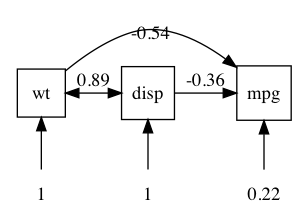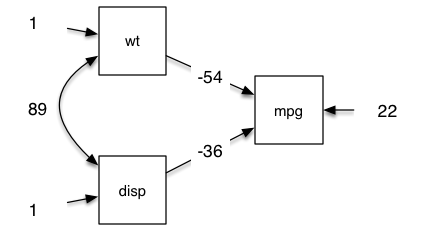# umx!

## plotting

TODO: Update for the ability to control plot layout with same, spline, max

# “The plot thickens”

If you’ve used `R`, you’ll be used to `plot` for graphical output. With umx, `plot` works for `umxRAM` and twin (`umxACE` etc.) models!

Just `plot(model)`, and you will get a path diagram displayed in your browser (or RStudio graph window if you use RStudio). We’ll learn later how to open plots in other applications (like Graphviz), including for editing in apps like OmniGraffle).

``````m1 = umxRAM("cars", data = mtcars, type="cov",
umxPath(c("wt", "disp"), to = "mpg"),
umxPath("wt", with = "disp"),
umxPath(var = c("wt", "disp", "mpg"))
)
plot(m1)
```````plot` writes a “dot” file. This is another Bell Labs invention (just like R’s predecessor “S”) to specify “graphs” -for our purposes, objects (nodes) linked by lines (“edges”)- and lay them out (not an easy problem). By default, it opens courtesy of Richard Iannone’s fabulous DiagrammR package.

## Inside a Graphviz file

Just so you know what we are working with, here’s the `.gv` file for the model above:

``````digraph G {

mpg  [shape = square];
wt   [shape = square];
disp [shape = square];
mpg_var  [label="0.22", shape = plaintext];
wt_var   [label="1",    shape = plaintext];
disp_var [label="1",    shape = plaintext];

# Single arrow paths
wt   -> mpg [label="-0.54"];
disp -> mpg [label="-0.36"];

# Variances
mpg_var  -> mpg;
wt_var   -> wt;
wt       -> disp [dir=both, label="0.89"];
disp_var -> disp;

{rank = same; mpg wt disp};
{rank = max ; mpg_var wt_var disp_var};

}
``````

You can manually edit this (it’s just text) to get a different layout.

On Mac, `plot` will open this file in your default app for .gv files. I set this to graphviz.

On MacOS `Omnigraffle` can open `.gv` files, and allows awesome publication-quality graphics.

So we can make the above example into this in about 1 minute (I timed it):## Parameters for plot.MxModel()

The parameters of plot give you a lot of flexibility. You can set the name of the file (it defaults to the model name), show unstandardised output, set the rounding for loadings, choose to show labels rather than estimates of paths, show or hide fixed paths, and means, and error variance.

``````plot(model, std = F, means = T, digits = 2, file = "name", ...)

``````

More advanced controls include showing labels, not showing fixed paths, or modifying how residuals are drawn with `resid = c("circle", "line", "none")`

These are described below.

#### Standardized output and digit rounding

You can optionally output standardised output using `std = TRUE`, and control the number of values after the decimal with `digits = ` (as well as `strip_zero = TRUE` to strip off leading “0.” from parameter values - can be helpful for cluttered figures).

``````plot(m1, std = TRUE, digits = 3, strip_zero = TRUE)
``````

#### Plot style: residuals, means, fixed paths

The correct way to draw a residual is a double headed arrow from an object back to the object. This is the default for `plot`.

Omnigraffle doesn’t import or allow you to edit these easily, so for omnigraffle editing, you might switch to resid = “line”.

For clarity you can turn this off all together with `resid = "none"`. You can also suppress drawing `means`, and turn on drawing `fixed` paths as you choose.

``````resid = c("circle", "line", "none")
fixed = FALSE,
means = FALSE,
``````

You can control how lines are drawn with the “splines” option. Users can also force the plot engine to group sets of variables at the top (`min`=), `same` or bottom (`max`) of the plot, which helps layout complex models.

#### File output and filename

The path model can be written to a file (the default is the name of the model with “.gv” suffix.

Don’t write any file

``````plot(m1, file = NA)
``````

Override the default name

``````plot(m1, file = "my nice example")

``````

Give a full file path

``````plot(m1, file = "~/tmp.gv") # specify a file

``````

TODO: tutorial on `umx_set_plot_file_suffix` and `umx_set_plot_format`

TODO: examples for `same` and `spline` parameters.